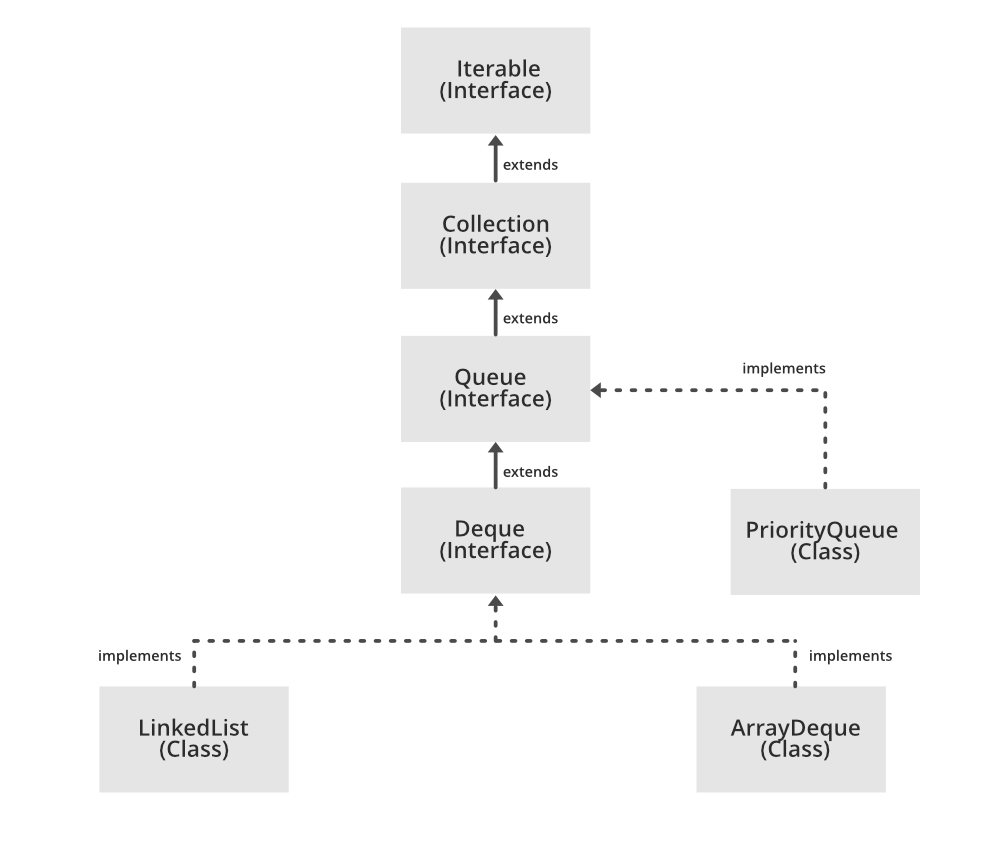GeeksforGeeks App
Open AppBrowser
Continue

# Using PriorityQueue to Have Sorted Collection of Data in Java

The Java util package has a queue interface that defines the queue interface which has methods defined for methods in a queue. The general implementation of a queue is a first-in-first-out ordering of elements. The interface has many methods we are focusing on two

• poll() – Retrieves and removes the head of this queue, or returns null if this queue is empty.
• offer(element e) – Inserts the specified element into this queue if it is possible to do so immediately without violating capacity restrictions.

The queue interface has many implementations. For special ordering within the queue, java introduces an implementation class Priority_Queue to allow ordering within the queue to be of a special type.

### Priority Queue

An unbounded priority queue based on a priority heap. The elements of the priority queue are ordered according to their natural ordering, or by a Comparator provided at queue construction time, depending on which constructor is used. A priority queue does not permit null elements. A priority queue relying on natural ordering also does not permit the insertion of non-comparable objects (doing so may result in ClassCastException).## Sorting elements using a Priority Queue

Let’s walk through some code examples of how a priority queue can be used to order elements in ascending or descending order.

### 1. Sorting elements in ascending order in the priority queue

A comparator class allows us to define how elements are ordered in the queue. To sort the elements in ascending order we need to define the queue as below. (The comparator has been written in an expanded form for simplicity.)

## Java

 `import` `java.util.Comparator;``import` `java.util.PriorityQueue;``import` `java.util.Queue;`` ` `public` `class` `Application {`` ` `    ``public` `static` `void` `main(String[] args) {``        ``Comparator ascendingOrderComparator = ``new` `Comparator() {``            ``@Override``            ``public` `int` `compare(Integer o1, Integer o2) {``                ``if` `(o1 < o2)``                    ``return` `-``1``;``                ``if` `(o1 == o2)``                    ``return` `0``;``                ``return` `1``;``            ``}``        ``};``        ``Queue priorityQueue = ``new` `PriorityQueue<>(ascendingOrderComparator);`` ` `        ``// insert elements in the queue``        ``priorityQueue.offer(``5``);``        ``priorityQueue.offer(``100``);``        ``priorityQueue.offer(``1``);``        ``priorityQueue.offer(``2``);`` ` `        ``// output``        ``while` `(!priorityQueue.isEmpty()) {``            ``System.out.print(priorityQueue.poll() + ``" "``);``        ``}``    ``}``}`

Output

`1 2 5 100 `

### 2. Sorting elements in descending order in the priority queue

A comparator class allows us to define how elements are ordered in the queue. To sort the elements in descending order we need to define the queue as below. (The comparator has been written in an expanded form for simplicity).

## Java

 `import` `java.util.Comparator;``import` `java.util.PriorityQueue;``import` `java.util.Queue;`` ` `public` `class` `Application {`` ` `    ``public` `static` `void` `main(String[] args) {``        ``Comparator descendingOrderComparator = ``new` `Comparator() {``            ``@Override``            ``public` `int` `compare(Integer o1, Integer o2) {``                ``if` `(o1 < o2)``                    ``return` `1``;``                ``if` `(o1 == o2)``                    ``return` `0``;``                ``return` `-``1``;``            ``}``        ``};``        ``Queue priorityQueue = ``new` `PriorityQueue<>(descendingOrderComparator);`` ` `        ``// insert elements in the queue``        ``priorityQueue.offer(``5``);``        ``priorityQueue.offer(``100``);``        ``priorityQueue.offer(``1``);``        ``priorityQueue.offer(``2``);`` ` `        ``// output``        ``while` `(!priorityQueue.isEmpty()) {``            ``System.out.print(priorityQueue.poll() + ``" "``);``        ``}``    ``}``}`

Output

`100 5 2 1 `

### 3. Sorting a collection of objects using a priority queue

We can sort a collection of objects based on a field of the object. Let’s take an example of a Person class which can be sorted by the age of the person in ascending order

## Java

 `import` `java.util.Comparator;``import` `java.util.PriorityQueue;``import` `java.util.Queue;`` ` `public` `class` `Application {``    ``public` `static` `void` `main(String[] args) {``        ``Comparator ascendingOrderComparator = ``new` `Comparator() {``            ``@Override``            ``public` `int` `compare(Person o1, Person o2) {``                ``if` `(o1.getAge() < o2.getAge())``                    ``return` `-``1``;``                ``if` `(o1.getAge() == o2.getAge())``                    ``return` `0``;``                ``return` `1``;``            ``}``        ``};``        ``Queue priorityQueue = ``new` `PriorityQueue<>(ascendingOrderComparator);``      ` `        ``// insert elements in the queue``        ``priorityQueue.offer(``new` `Person(``"john"``, ``"doe"``, ``5``));``        ``priorityQueue.offer(``new` `Person(``"karan"``, ``"agarwal"``, ``100``));``        ``priorityQueue.offer(``new` `Person(``"anon"``, ``"test"``, ``1``));``        ``priorityQueue.offer(``new` `Person(``"jien"``, ``"wang"``, ``2``));``         ` `        ``// output``        ``while` `(!priorityQueue.isEmpty()) {``            ``System.out.print(priorityQueue.poll() + ``" "``);``        ``}``    ``}``}`` ` `class` `Person {``    ``private` `String firstName;``    ``private` `String lastName;``    ``private` `int` `age;`` ` `    ``public` `Person(String firstName, String lastName, ``int` `age) {``        ``this``.firstName = firstName;``        ``this``.lastName = lastName;``        ``this``.age = age;``    ``}`` ` `    ``public` `int` `getAge() {``        ``return` `age;``    ``}`` ` `    ``@Override``    ``public` `String toString() {``        ``return` `"Person{"` `+``                ``"firstName='"` `+ firstName + '\``''` `+``                ``", lastName='"` `+ lastName + '\``''` `+``                ``", age="` `+ age +``                ``'}'``;``    ``}``}`

Output

```Person{firstName='anon', lastName='test', age=1}
Person{firstName='jien', lastName='wang', age=2}
Person{firstName='john', lastName='doe', age=5}
Person{firstName='karan', lastName='agarwal', age=100} ```

My Personal Notes arrow_drop_up